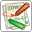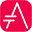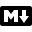# nwdiagnwdiag has been created by Takeshi Komiya and allows to quickly draw network diagrams. So we thank him for his creation!

Since the syntax is clear and simple, this has been integrated within PlantUML. We reuse here the examples that Takeshi has documented.

## Simple diagram```@startuml nwdiag { network dmz { address = "210.x.x.x/24" web01 [address = "210.x.x.1"]; web02 [address = "210.x.x.2"]; } network internal { address = "172.x.x.x/24"; web01 [address = "172.x.x.1"]; web02 [address = "172.x.x.2"]; db01; db02; } } @enduml ```## define multiple addresses```@startuml nwdiag { network dmz { address = "210.x.x.x/24" // set multiple addresses (using comma) web01 [address = "210.x.x.1, 210.x.x.20"]; web02 [address = "210.x.x.2"]; } network internal { address = "172.x.x.x/24"; web01 [address = "172.x.x.1"]; web02 [address = "172.x.x.2"]; db01; db02; } } @enduml ```## grouping nodesNot fully implemented yet

```@startuml nwdiag { network Sample_front { address = "192.168.10.0/24"; // define group group web { web01 [address = ".1"]; web02 [address = ".2"]; } } network Sample_back { address = "192.168.20.0/24"; web01 [address = ".1"]; web02 [address = ".2"]; db01 [address = ".101"]; db02 [address = ".102"]; // define network using defined nodes group db { db01; db02; } } } @enduml ``````@startuml nwdiag { // define group at outside network definitions group { color = "#FF7777"; web01; web02; db01; } network dmz { web01; web02; } network internal { web01; web02; db01; } } @enduml ```## Extended Syntax```@startuml nwdiag { network Sample_front { address = "192.168.10.0/24" color = "red" // define group group web { web01 [address = ".1", shape = "node"] web02 [address = ".2"] } } network Sample_back { address = "192.168.20.0/24" web01 [address = ".1"] web02 [address = ".2"] db01 [address = ".101", shape = database ] db02 [address = ".102"] // define network using defined nodes group db { db01; db02; } } } @enduml ```## peer networks```@startuml nwdiag { inet [shape = cloud]; inet -- router; network { router; web01; web02; } } @enduml ```